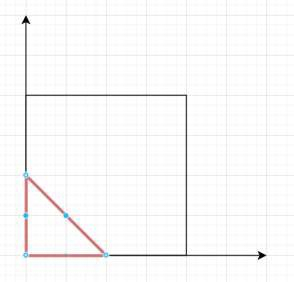# Largest Triangle Area in Python

Suppose we have a list of points on a plane. We have to find the area of the largest triangle that can be formed by any 3 of the points.

So, if the input is like [[0,0],[0,1],[1,0],[0,2],[2,0]], then the output will be 2To solve this, we will follow these steps −

• res := 0
• N := size of points list
• for i in range 0 to N - 2, do
• for j in range i + 1 to N - 1, do
• for k in range i + 2 to N, do
• (x1, y1) := points[i],
• (x2, y2) := points[j],
• (x3, y3) := points[k]
• res := maximum of res, 0.5 * |x1 *(y2 - y3) + x2 *(y3 - y1) + x3 *(y1 - y2)
• return res

Let us see the following implementation to get better understanding −

## Example

Live Demo

class Solution:
def largestTriangleArea(self, points):
res = 0
N = len(points)
for i in range(N - 2):
for j in range(i + 1, N - 1):
for k in range(i + 2, N):
(x1, y1), (x2, y2), (x3, y3) = points[i],points[j],points[k]
res = max(res, 0.5 * abs(x1 * (y2 - y3) + x2 * (y3 - y1) + x3 * (y1 - y2)))
return res
ob = Solution()
print(ob.largestTriangleArea([[0,0],[0,1],[1,0],[0,2],[2,0]]))

## Input

[[0,0],[0,1],[1,0],[0,2],[2,0]]

## Output

2.0